# MATLAB Fundamentals: Vectorization

A coworker whose background is in embedded systems (with a C background and no MATLAB at all), after hearing my rants that people are coding MATLAB like C using way more for-loops than necessary, asked me if he has two vectors,

a = 0:32767;
b = 0:32767;

and he want all combinations of the elements in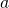and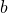so that for each index pair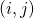, he will get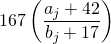There are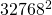combinations out there. At first, I showed him the typical method shown in the MATLAB’s introduction materials:

% Should have used ndgrid() for a more natural (column first) layout
[B, A] = meshgrid(a, b);

C = 167*(A+42)./(B+17)

Then he asked, ‘This way I have to store the matrices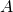and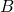. Wouldn’t it be memory intensive? Is there a better way to do it like with functional programming?’ Now I have to show him a more advanced trick that requires some mental leaps (the ones necessary to get sophisticated at the MATLAB language):

C = 167*bsxfun(@rdivide, a'+42, b+17)

This one liner does not save intermediate input values, so it’s memory efficient as well.

bsxfun() is a function that takes two inputs (we call it a binary function) which any of them can be a matrix, vector or scalar. It will conceptually expand the dimensions so the function handle (e.g. @rdivide) get to apply to all combinations as if the inputs are expanded (repeated) to the longer of each dimension supplied. I bet under the hood it’s just a pair of for-loops with the loop increments managed so it doesn’t waste memory storing the intermediaries.

In the example above, I have a column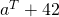and a row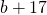. The output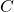is arranged as ifis copied right to meet the length of, andis copied down to meet the length of.

This involves two major concepts one needs to program the MATLAB way : vectorization and anonymous functions. Not something you’d tell a day-zero beginner (probably scare them off), but showing them a Ninja trick after they understand the beginner’s method might motivate them to learn the true power of MATLAB.

Rik has pointed out in the comments below that TMW introduced implicit expansion that makes the bsxfun() obsolete. This is an excellent move (readability), despite it won’t police matrix crimes anymore so educators should teach about implicit expansion as the first thing as it’d be hard to debug is the user is not aware of the behavior. Thanks Rik!

1,107 total views,  1 views today0 Followers

Most reacted commentRecent comment authors